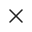# 数据监听器 observers

## 使用说明

``````Component({
attached() {
this.setData({
numberA: 1,
numberB: 2,
});
},
observers: {
// 在更新 this.data.numberA 或 this.data.numberB 时，执行这个函数
"numberA, numberB"(numberA, numberB) {
this.setData({
sum: numberA + numberB,
});
},
},
});``````

## 监听语法

`observers` 是 object 类型，它的 key 是监听表达式，value 是触发监听的回调函数，监听表达式的类型如下：

`.` 分隔的路径表达式`a.b.c` 表示监听 `this.data.a.b.c`

`,` 分隔的多路径表达式`a, b` 表示同时监听 `this.data.a``this.data.b`

• `a, b` 的监听回调依次传入 `this.data.a`, `this.data.b` 两个参数；
• `a.**` 的监听回调传入的参数是 `this.data.a`
• `**` 的监听回调传入的参数是 `this.data`

## observers 的触发时机

• 只有属性更新或通过 `setData` 更新才可能触发数据监听器，直接修改 data 如 `this.data.xxx = 'xxx'` 不会触发数据监听器；
• 如果监听的是自定义组件 `properties` 定义的属性，父组件初始化时给子组件的被监听的属性赋值，也会触发监听回调；
• 如果 `setData` 涉及的数据字段的值没有发生变化（与旧值做深比较），则它们不会触发数据监听器；
• 如果 `properties``observers` 同时定义了对同一个数据字段的监听回调，触发顺序是先触发 `observers` 的回调，再触发 `properties` 中定义的回调。

## 代码示例

``````Component({
observers: {
"some.subfield"(subfield) {
// this.data.some.subfield 或 this.data.some 被改变时触发
console.log(subfield === this.data.some.subfield); // true
},
"arr"(arr12) {
// this.data.arr 或 this.data.arr 被改变时触发
console.log(arr12 === this.data.arr);// true
},
"some.field.**"(field) {
// this.data.some.field 或其下任何子数据字段或this.data.some 被改变时触发
console.log(field === this.data.some.field);// true
},
"**"(field) {
// 每次数据被改变时都触发
console.log(field === this.data);// true
},
},
attached() {
// 这样会触发 some.field.** 的 observer
this.setData({
"some.field": {
/* ... */
},
});
// 这样也会触发 some.field.** 的 observer
this.setData({
"some.field.xxx": {
/* ... */
},
});
// 这样还是会触发 some.field.** 的 observer
this.setData({
some: {
/* ... */
},
});
});``````

## Bug & Tip

• Tip: 如果在数据监听器函数中使用 `setData` 设置本身监听的数据字段，可能会导致死循环，需要特别留意。